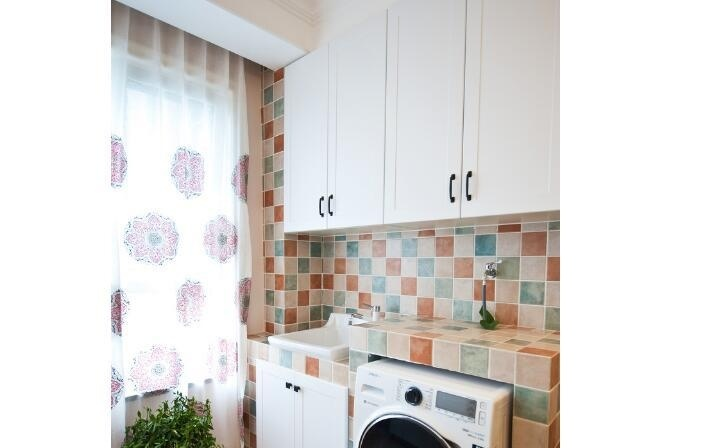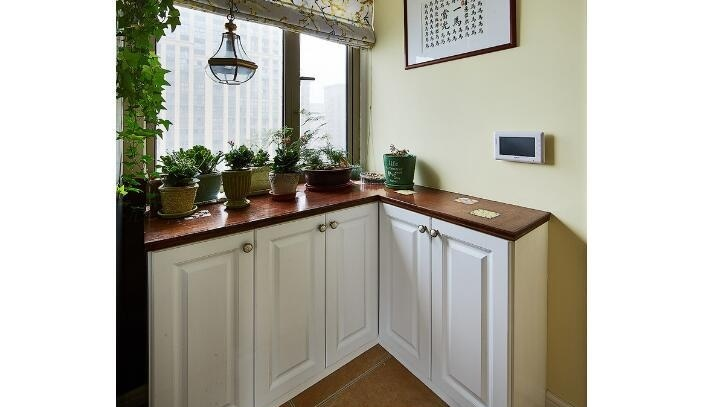|

# 阳台储物柜尺寸 阳台储物柜用什么材料好

阳台是我们在装修的时候不能忽视的一项工程，我们在设计阳台的时候往往喜欢在阳台上放置一款阳台储物柜，一款尺寸合适的阳台储物柜不仅比较美观，储物能力也比较强。那么一般来说阳台储物柜的尺寸是多少呢，阳台储物柜哪种材料比较好呢，下面我们就一起来了解一下吧。阳台储物柜尺寸 阳台储物柜用什么材料好

阳台储物柜尺寸

随着这社会的发展现在储物柜已经不再是以前最初的那种随便拿个箱子就行了的。现在的储物柜已经是我们的家具之一，可以说是我们居家生活中必不可少的一部分。所以在购置储物柜的时候针对我们所要存放的东西而需要十分的注意这储物柜尺寸的大小。现在我们看见的这款储物柜尺寸规格是十分的合理的600*450*1100mm。这款储物柜所采用是高档的实木木材。整体上采用的是五抽设计，储存空间十分的合理。这种地中海风格的储物柜更加的凸显出这款储物柜师傅呢的平衡和谐。不管是放置在家中的任何一个地方也能为我们的居家生活带来阵阵的淡雅清新之感。这款储物柜尺寸大小也是刚刚好。一提到这储物柜尺寸很多的人在购买的时候更加的偏向于这中精致大气，同时对这储物柜的储物量大家也是希望能最大化。这我们现在所看见的这一款储物柜尺寸十分的大整个的储存空间也大。外观也是十分精美，其采用的是进口实木所制。质地是分的坚硬十分的耐用，这一款储物柜采用的是经典的古典色彩，显得整体上十分的大气古朴。再加上这款储物柜的外表纹路十分的清晰美观。显得十分的富有内涵。线条采用的是流畅的线条处理。其边缘十分的圆润大方。使用中的安全系数也是十分的高。像这一款储物柜尺寸为975*510*1255mm。是不是这款储物柜尺寸十分的大呢？能储存更多的东西。阳台储物柜参考价格

韩菲尔韩式五格阳台抽屉式储物柜

参考价格：￥1700

材质：板式+实木

推荐理由：这款五格储物柜设计得简洁大方，主要以清新田园风为设计主线，平衡、和谐、温暖的韩式田园风格，为你的家居生活吹来阵阵清新恬淡的清风，让你的生活更加贴近自然，带您领略不一样的田园家居生活。

凯撒豪庭美式五格阳台抽屉式储物柜

参考价格：￥1500

材质：板式+实木

推荐理由：拥有简约的外观设计，深色调带点成熟与大气，全部线条与花饰由人工雕刻而成，柜面的弧线形结构呈波浪形，为居家生活带来更多的浪漫气息。这就是美式古典的储物柜。

韩菲尔粉甜法式卧室家具五格储物柜

参考价格：￥1960

材质：板式+实木

推荐理由：这套储物柜拥有精湛的制作工艺，精致的雕花更为其增添一分独特的美丽，更加显得精致而有特色，加上其淡雅的色泽，整体酝酿出一款时尚而富有新意的家具，为你营造一个高品质的生活环境。

此外，无论哪种储物柜板材，都要注意其环保性能，加工中难免会有胶水等粘合剂使用，散发的甲醛是家具污染的主要问题，消费者一定要引起重视。阳台抽屉式储物柜需要定期清理，保持室内居家环境的整洁，用湿抹布将储物柜抹干净，用干抹布将其抹干，避免水分的残留，以免腐蚀储物柜；避免储物柜接触尖锐的物品，最好选用布制较为柔软的布料擦洗；避免腐蚀性气体和液体接触。阳台储物柜用什么材料好

1、实木

实木材质的阳台储物柜的质地是非常的坚硬，而且它的使用寿命也相对比较长久，并且很耐撞。而实木的种类也有很多，不同种类特性也不同，檀木、胡桃木等等都是比较常见的。大家在选择之前都要做好充分的了解，才可以挑选到质量好的储物柜。

2、铁质

铁质的阳台储物柜也是比较坚固的，而且成本并不高，价格很实惠，有一定的防水作用，而且相对于实木材质的储物柜来说比较防虫。但是在家庭阳台使用的相对比较少，在学生宿舍、职工宿舍这种集体环境中使用的情况比较多。

3、塑料

塑料阳台储物柜采用聚乙烯或者聚丙烯制造，价格比较便宜，密封性好，而且耐油污没有异味。非常容易清洁，用起来也十分方便，容易收拾，节省了空间。但同时塑料也比较容易氧化，要注意温度不要过高，而且塑料不是很结实，要注意不要剧烈撞击。

`声明：本文由入驻焦点开放平台的作者撰写，除焦点官方账号外，观点仅代表作者本人，不代表焦点立场错误信息举报电话： 400-099-0099，邮箱：jubao@vip.sohu.com，或点此进行意见反馈，或点此进行举报投诉。`A B C D E F G H J K L M N P Q R S T W X Y Z
A - B - C - D - E
• A
• 鞍山
• 安庆
• 安阳
• 安顺
• 安康
• 澳门
• B
• 北京
• 保定
• 包头
• 巴彦淖尔
• 本溪
• 蚌埠
• 亳州
• 滨州
• 北海
• 百色
• 巴中
• 毕节
• 保山
• 宝鸡
• 白银
• 巴州
• C
• 承德
• 沧州
• 长治
• 赤峰
• 朝阳
• 长春
• 常州
• 滁州
• 池州
• 长沙
• 常德
• 郴州
• 潮州
• 崇左
• 重庆
• 成都
• 楚雄
• 昌都
• 慈溪
• 常熟
• D
• 大同
• 大连
• 丹东
• 大庆
• 东营
• 德州
• 东莞
• 德阳
• 达州
• 大理
• 德宏
• 定西
• 儋州
• 东平
• E
• 鄂尔多斯
• 鄂州
• 恩施
F - G - H - I - J
• F
• 抚顺
• 阜新
• 阜阳
• 福州
• 抚州
• 佛山
• 防城港
• G
• 赣州
• 广州
• 桂林
• 贵港
• 广元
• 广安
• 贵阳
• 固原
• H
• 邯郸
• 衡水
• 呼和浩特
• 呼伦贝尔
• 葫芦岛
• 哈尔滨
• 黑河
• 淮安
• 杭州
• 湖州
• 合肥
• 淮南
• 淮北
• 黄山
• 菏泽
• 鹤壁
• 黄石
• 黄冈
• 衡阳
• 怀化
• 惠州
• 河源
• 贺州
• 河池
• 海口
• 红河
• 汉中
• 海东
• I
• J
• 晋中
• 锦州
• 吉林
• 鸡西
• 佳木斯
• 嘉兴
• 金华
• 景德镇
• 九江
• 吉安
• 济南
• 济宁
• 焦作
• 荆门
• 荆州
• 江门
• 揭阳
• 金昌
• 酒泉
• 嘉峪关
K - L - M - N - P
• K
• 开封
• 昆明
• 昆山
• L
• 廊坊
• 临汾
• 辽阳
• 连云港
• 丽水
• 六安
• 龙岩
• 莱芜
• 临沂
• 聊城
• 洛阳
• 漯河
• 娄底
• 柳州
• 来宾
• 泸州
• 乐山
• 六盘水
• 丽江
• 临沧
• 拉萨
• 林芝
• 兰州
• 陇南
• M
• 牡丹江
• 马鞍山
• 茂名
• 梅州
• 绵阳
• 眉山
• N
• 南京
• 南通
• 宁波
• 南平
• 宁德
• 南昌
• 南阳
• 南宁
• 内江
• 南充
• P
• 盘锦
• 莆田
• 平顶山
• 濮阳
• 攀枝花
• 普洱
• 平凉
Q - R - S - T - W
• Q
• 秦皇岛
• 齐齐哈尔
• 衢州
• 泉州
• 青岛
• 清远
• 钦州
• 黔南
• 曲靖
• 庆阳
• R
• 日照
• 日喀则
• S
• 石家庄
• 沈阳
• 双鸭山
• 绥化
• 上海
• 苏州
• 宿迁
• 绍兴
• 宿州
• 三明
• 上饶
• 三门峡
• 商丘
• 十堰
• 随州
• 邵阳
• 韶关
• 深圳
• 汕头
• 汕尾
• 三亚
• 三沙
• 遂宁
• 山南
• 商洛
• 石嘴山
• T
• 天津
• 唐山
• 太原
• 通辽
• 铁岭
• 泰州
• 台州
• 铜陵
• 泰安
• 铜仁
• 铜川
• 天水
• 天门
• W
• 乌海
• 乌兰察布
• 无锡
• 温州
• 芜湖
• 潍坊
• 威海
• 武汉
• 梧州
• 渭南
• 武威
• 吴忠
• 乌鲁木齐
X - Y - Z
• X
• 邢台
• 徐州
• 宣城
• 厦门
• 新乡
• 许昌
• 信阳
• 襄阳
• 孝感
• 咸宁
• 湘潭
• 湘西
• 西双版纳
• 西安
• 咸阳
• 西宁
• 仙桃
• 西昌
• Y
• 运城
• 营口
• 盐城
• 扬州
• 鹰潭
• 宜春
• 烟台
• 宜昌
• 岳阳
• 益阳
• 永州
• 阳江
• 云浮
• 玉林
• 宜宾
• 雅安
• 玉溪
• 延安
• 榆林
• 银川
• Z
• 张家口
• 镇江
• 舟山
• 漳州
• 淄博
• 枣庄
• 郑州
• 周口
• 驻马店
• 株洲
• 张家界
• 珠海
• 湛江
• 肇庆
• 中山
• 自贡
• 资阳
• 遵义
• 昭通
• 张掖
• 中卫

1室1厅1厨1卫1阳台

1
2
3
4
5

0
1
2

1

1

0
1
2
3报名成功，资料已提交审核A B C D E F G H J K L M N P Q R S T W X Y Z
A - B - C - D - E
• A
• 鞍山
• 安庆
• 安阳
• 安顺
• 安康
• 澳门
• B
• 北京
• 保定
• 包头
• 巴彦淖尔
• 本溪
• 蚌埠
• 亳州
• 滨州
• 北海
• 百色
• 巴中
• 毕节
• 保山
• 宝鸡
• 白银
• 巴州
• C
• 承德
• 沧州
• 长治
• 赤峰
• 朝阳
• 长春
• 常州
• 滁州
• 池州
• 长沙
• 常德
• 郴州
• 潮州
• 崇左
• 重庆
• 成都
• 楚雄
• 昌都
• 慈溪
• 常熟
• D
• 大同
• 大连
• 丹东
• 大庆
• 东营
• 德州
• 东莞
• 德阳
• 达州
• 大理
• 德宏
• 定西
• 儋州
• 东平
• E
• 鄂尔多斯
• 鄂州
• 恩施
F - G - H - I - J
• F
• 抚顺
• 阜新
• 阜阳
• 福州
• 抚州
• 佛山
• 防城港
• G
• 赣州
• 广州
• 桂林
• 贵港
• 广元
• 广安
• 贵阳
• 固原
• H
• 邯郸
• 衡水
• 呼和浩特
• 呼伦贝尔
• 葫芦岛
• 哈尔滨
• 黑河
• 淮安
• 杭州
• 湖州
• 合肥
• 淮南
• 淮北
• 黄山
• 菏泽
• 鹤壁
• 黄石
• 黄冈
• 衡阳
• 怀化
• 惠州
• 河源
• 贺州
• 河池
• 海口
• 红河
• 汉中
• 海东
• I
• J
• 晋中
• 锦州
• 吉林
• 鸡西
• 佳木斯
• 嘉兴
• 金华
• 景德镇
• 九江
• 吉安
• 济南
• 济宁
• 焦作
• 荆门
• 荆州
• 江门
• 揭阳
• 金昌
• 酒泉
• 嘉峪关
K - L - M - N - P
• K
• 开封
• 昆明
• 昆山
• L
• 廊坊
• 临汾
• 辽阳
• 连云港
• 丽水
• 六安
• 龙岩
• 莱芜
• 临沂
• 聊城
• 洛阳
• 漯河
• 娄底
• 柳州
• 来宾
• 泸州
• 乐山
• 六盘水
• 丽江
• 临沧
• 拉萨
• 林芝
• 兰州
• 陇南
• M
• 牡丹江
• 马鞍山
• 茂名
• 梅州
• 绵阳
• 眉山
• N
• 南京
• 南通
• 宁波
• 南平
• 宁德
• 南昌
• 南阳
• 南宁
• 内江
• 南充
• P
• 盘锦
• 莆田
• 平顶山
• 濮阳
• 攀枝花
• 普洱
• 平凉
Q - R - S - T - W
• Q
• 秦皇岛
• 齐齐哈尔
• 衢州
• 泉州
• 青岛
• 清远
• 钦州
• 黔南
• 曲靖
• 庆阳
• R
• 日照
• 日喀则
• S
• 石家庄
• 沈阳
• 双鸭山
• 绥化
• 上海
• 苏州
• 宿迁
• 绍兴
• 宿州
• 三明
• 上饶
• 三门峡
• 商丘
• 十堰
• 随州
• 邵阳
• 韶关
• 深圳
• 汕头
• 汕尾
• 三亚
• 三沙
• 遂宁
• 山南
• 商洛
• 石嘴山
• T
• 天津
• 唐山
• 太原
• 通辽
• 铁岭
• 泰州
• 台州
• 铜陵
• 泰安
• 铜仁
• 铜川
• 天水
• 天门
• W
• 乌海
• 乌兰察布
• 无锡
• 温州
• 芜湖
• 潍坊
• 威海
• 武汉
• 梧州
• 渭南
• 武威
• 吴忠
• 乌鲁木齐
X - Y - Z
• X
• 邢台
• 徐州
• 宣城
• 厦门
• 新乡
• 许昌
• 信阳
• 襄阳
• 孝感
• 咸宁
• 湘潭
• 湘西
• 西双版纳
• 西安
• 咸阳
• 西宁
• 仙桃
• 西昌
• Y
• 运城
• 营口
• 盐城
• 扬州
• 鹰潭
• 宜春
• 烟台
• 宜昌
• 岳阳
• 益阳
• 永州
• 阳江
• 云浮
• 玉林
• 宜宾
• 雅安
• 玉溪
• 延安
• 榆林
• 银川
• Z
• 张家口
• 镇江
• 舟山
• 漳州
• 淄博
• 枣庄
• 郑州
• 周口
• 驻马店
• 株洲
• 张家界
• 珠海
• 湛江
• 肇庆
• 中山
• 自贡
• 资阳
• 遵义
• 昭通
• 张掖
• 中卫• 手机• 分享
• 设计
免费设计
• 计算器
装修计算器
• 入驻
合作入驻
• 联系
联系我们
• 置顶
返回顶部